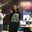Trusted answers to developer questions
Trusted Answers to Developer Questions

Related Tags

# Introduction to nondeterministic finite automatonAyesha Kanwal

Grokking Modern System Design Interview for Engineers & Managers

Ace your System Design Interview and take your career to the next level. Learn to handle the design of applications like Netflix, Quora, Facebook, Uber, and many more in a 45-min interview. Learn the RESHADED framework for architecting web-scale applications by determining requirements, constraints, and assumptions before diving into a step-by-step design process.

### Overview

nondeterministic finite automaton has one or more than one transition from one state to another or itself.

An NFA requires less space than a DFAdeterministic finite automaton for its construction. Moreover, a trap stateA state from which a transition can not escape. Also called a "dead state". is not required for an NFA.

### Components of an NFA

Assume an NFA for a language L with the five tuples $$ where

• $Q$ is the finite set of states.
• $Σ$ is the input symbols.
• $q_0$ is the start state.
• $δ$ is the transition function.
• $F$ is the final state.

It is to be noted that $δ: Q \times Σ \rightarrow 2 ^ Q$. This means that the next possible states belong to the power set of $Q$.

### Example

The following is an example of an NFA, where $q_0$ is the start state.

NFA

RELATED TAGS

CONTRIBUTORAyesha Kanwal